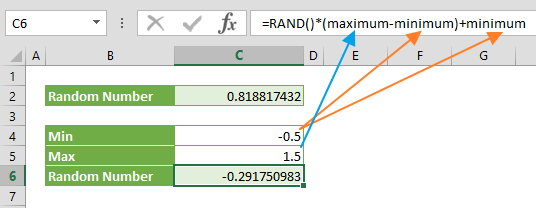The RAND function returns an evenly distributed random numbers greater than or equal to 0 and less than 1. In this guide, we’re going to show you how to use the RAND function and also go over some tips and error handling methods.

# Supported versions

• All Excel versions

RAND()

# Arguments

The RAND function doesn't require any arguments.

Examples

## Simple scenario

=RAND()
formula shows a basic use case of the RAND function. The function doesn't require any arguments. A random value will be regenerated every time the workbook calculations run. Press F9 to re-run calculations and update the value.## Generate between limits

The RAND function returns a number between 0 and 1, but the return value can never be 1. If you want to modify its limits use the following formula:

=RAND()*(maximum-minimum)+minimum

The minimum and maximum values are the limits. As a result, a formula like =RAND()*(1.5-(-0.5))+(-0.5) returns a random number between -0.5 and 1.5.# Tips

• The RAND function is a volatile function that returns a new random real number after every workbook calculation. Press F9 to update the value.
• Microsoft states Excel uses the Mersenne Twister algorithm (MT19937) to generate random numbers.
• Use the RANDBETWEEN function to return a random whole number between specified limits.
• To generate a set of random numbers, select the cells, enter the =RAND() formula, and press Ctrl + Enter (⌃ + Return in Mac).
• Microsoft has announced that they're soon going to release the RANDARRAY function to Office 365 subscribers. This new dynamic array function allows creating an array of randomly generated numbers.